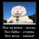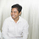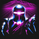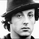50055瀏覽
50055
No scammers allowed. I protect CT

This dude on Twitter @TradingScript(UT Bot) tried to take this free script and sell it.

All the inputs he put in are there to make it look complex and don't actually do anything.

The only thing that changes is "Key Value". This is the ATR multiplier and in basic terms determines the sensitivity on the indicator. The original setting by HPotter is 3.5 multiplier (key vaule) and an ATR period of 5.

So yeah I recreated the script.

**If you compare this to that scammers script 95% of the signals are the same, I don't know the exact setting he used..
**HPotter is a Legend

Nov 02

Dec 06Hello my friend, I have a strategy that I want to do backtesting, could you give me a hand.
strategy:

BB = 2

BB length = 20

BB candle period = 300

SMA cross candles = 12

SMA candle period = 14400

SMA fast length = 50

SMA slow length = 200

EMA cross candles = 80
.
EMA candle period = 300

EMA fast length = 8

EMA slow length = 13

RSI candle period = 300

RSI length = 2

STOCH candle period = 300
STOCHRSI calculations
STOCH length = 5